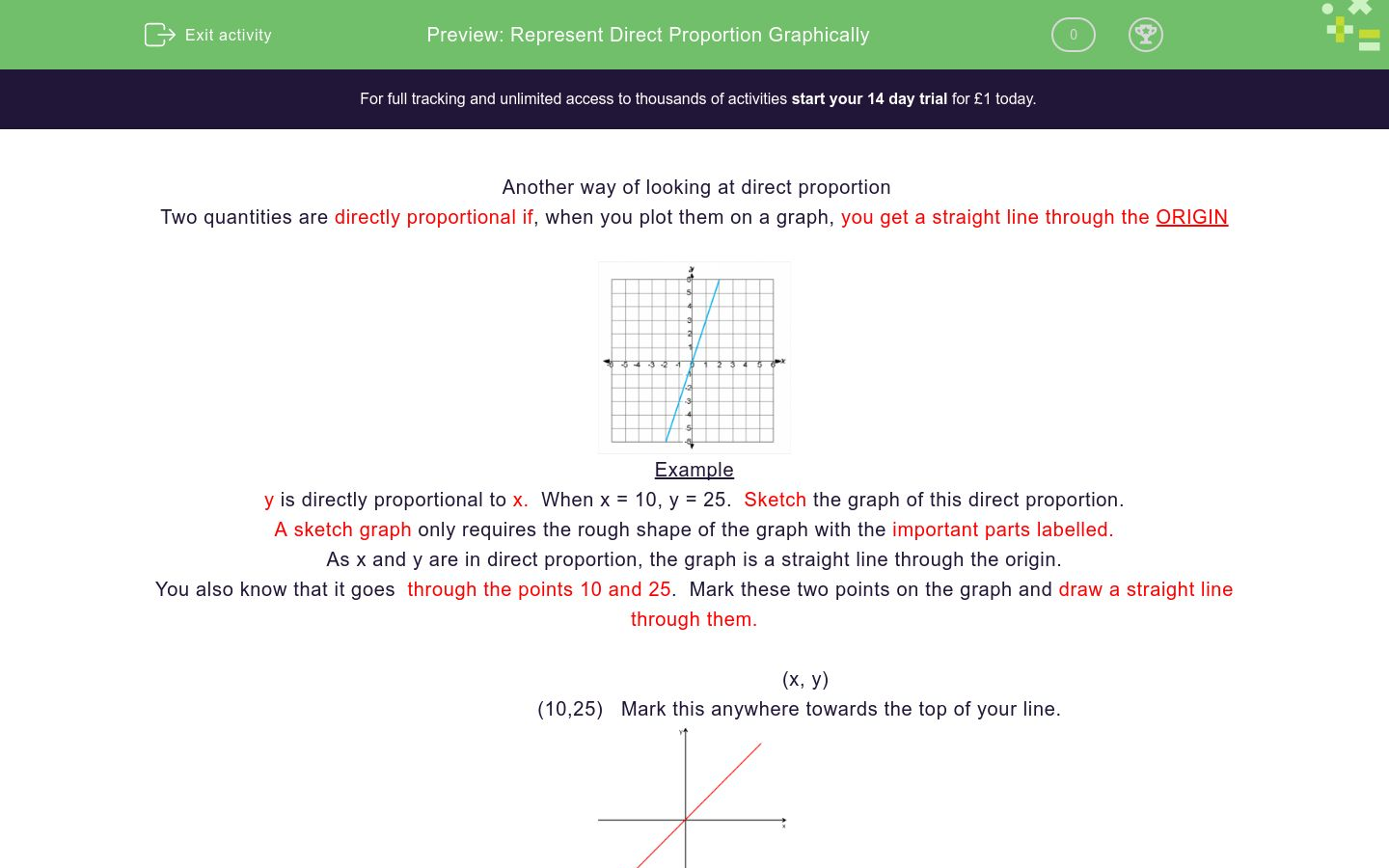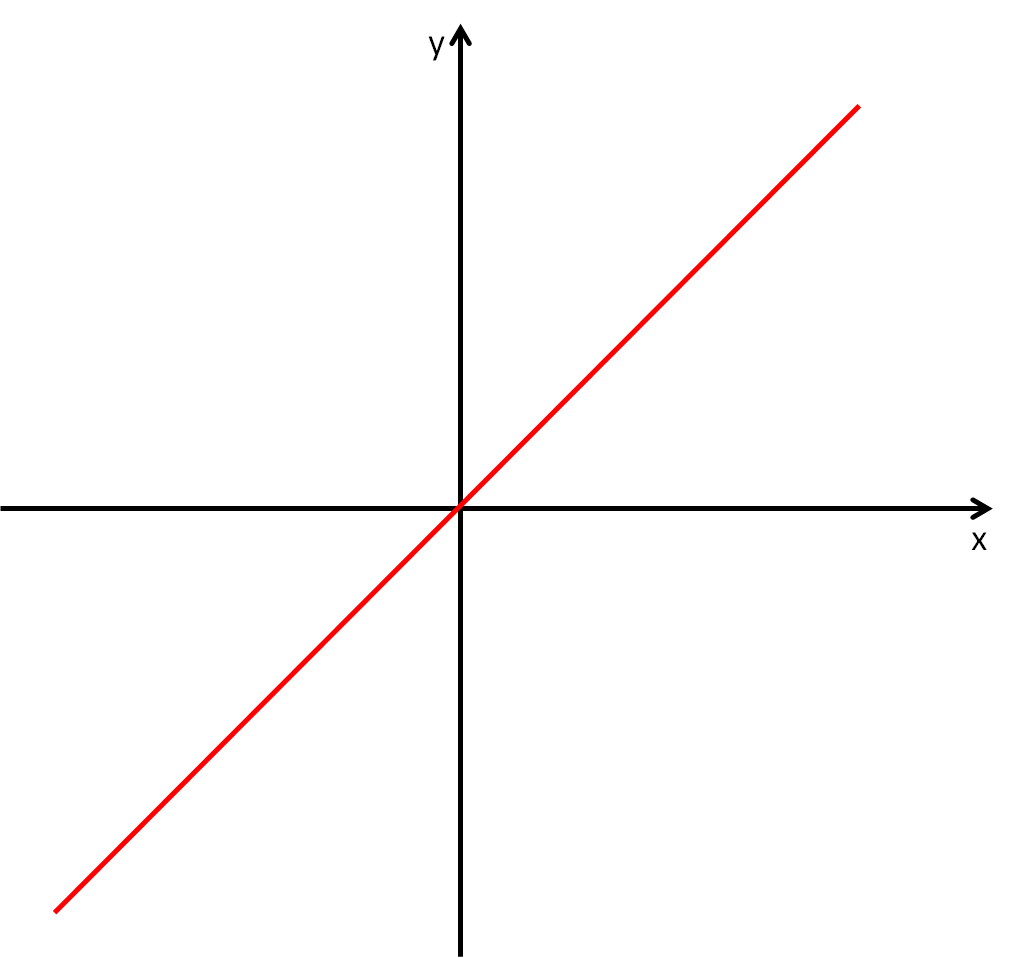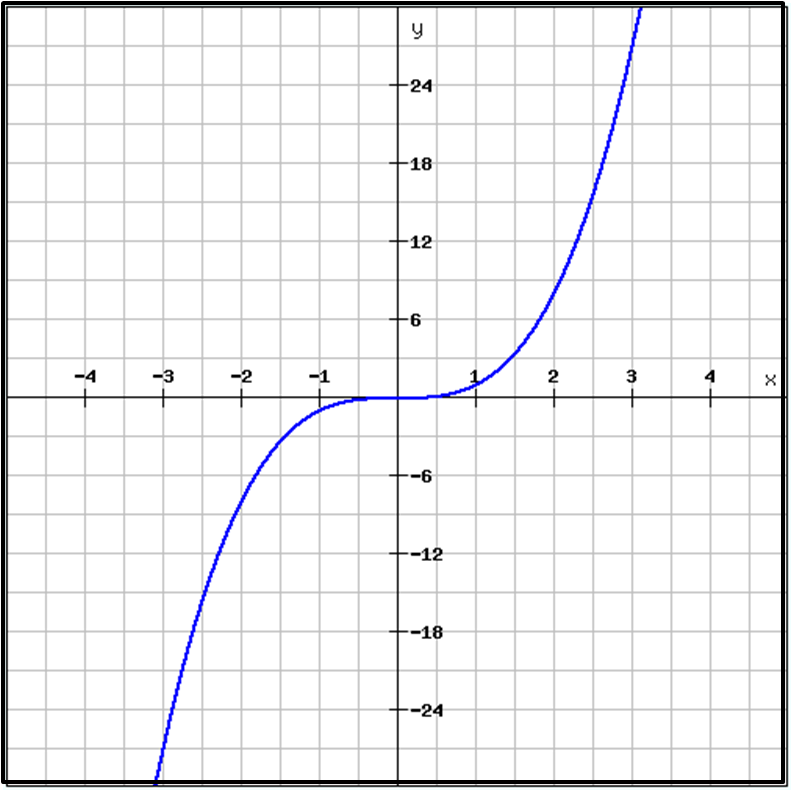# Represent Direct Proportion Graphically

In this worksheet, students will represent direct proportion graphically and solve real life problems.Key stage:  KS 4

GCSE Subjects:   Maths

GCSE Boards:   AQA, Eduqas, Pearson Edexcel, OCR

Curriculum topic:   Ratio, Proportion and Rates of Change

Curriculum subtopic:   Ratio, Proportion and Rates of Change, Direct and Inverse Proportion

Difficulty level:### QUESTION 1 of 10

Another way of looking at direct proportion

Two quantities are directly proportional if, when you plot them on a graph, you get a straight line through the ORIGINExample

y is directly proportional to x.  When x = 10, y = 25.  Sketch the graph of this direct proportion.

A sketch graph only requires the rough shape of the graph with the important parts labelled.

As x and y are in direct proportion, the graph is a straight line through the origin.

You also know that it goes  through the points 10 and 25.  Mark these two points on the graph and draw a straight line through them.

(x, y)

(10,25)   Mark this anywhere towards the top of your line.How easy is that?The other thing about graphs of direct proportion you would be asked to do is, work out the co-ordinates from given information.

y is directly proportional to x.  Complete the table

 x 3 5 10 12 y 25 100

Hurrah, we have been  given information to work from, which makes the task so much easier.

From that table we can see that x = 5, y = 25

Divide y by to find our faithful friend the multiplier

y = 25 ÷ 5 = 5

Multiply each value of x in the table by 5 to get the missing value of y

Divide the value of y by 5 to get the missing value of x

 x 3 5 10 12 100 ÷ 5 = 20 y 3 x 5= 15 25 10 x 5 = 50 12 x 5 =60 100

Once you have your values you can draw your graph.   Remember we are looking for a straight line.

If your line is a bit wonky, go back and check your table of values.  Don't try and disguise the line as being straight, I know it is tempting to do so.Hey presto..that is all there is to it.

Direct proportion graphs can be used to work out all sorts of things like, currency conversion and fuel consumption.

A                                                     B                                                            CWhich of these graphs are not direct proportion graphs?

A

B

C

Y is directly proportional to x.  Find out the missing value in the table to ensure your graph comes out as a straight line.

 x 8 12 y 2

6

4

14

3

Y is directly proportional to X.

Which y value is incorrect?

 x 2 7 10 12 y 6 22 30 36

y is directly proportional to x

 x -2 0 3 y -10 30

Before you check your answers draw the graph to ensure you have a straight line.

s is directly proportional to t.  When S = 80, t = 4.

Work out the value of  s when t = 13.

r is directly proportional to t.  When r = 9, t = 6.

What is the equation that represents this direct proportion?

Choose 3 numbers to represent t and use your multiplier to draw a graph.  Do you get a straight line?

t = 1.5r

r = 1.5t

r = 1.5

t = 1.5

p is directly proportional to q.  When p = 14, q = 4.

Find the value of p when q = 8.

These sets of co-ordinates are used to draw graphs of direct proportion.

Which set of co-ordinates are the odd one out?  Before you check your answer could you explain why?

A

 x 7 5 8 3 y 21 15 24 9

B

 x 1 2 4 6 y 2 3 5 9

C

 x 1 2 3 4 y 3 6 9 12
A

B

CYou can use direct proportion graphs to solve problems such as currency converting.

Peter wants to change  Pounds (£) into Dollars (\$) and vice versa.

The direct proportion of \$ to £ is shown in the table below

 £ 0 1 2 3 4 5 \$ 0 1.2 2.4 3.6 4.8 6

Use the table to plot the graph to convert pounds (£) into Dollars (\$).

Use your graph to convert £3.20 into dollars. (\$)

Use your graph to convert \$5 into pounds (£)

A

B

CRoger goes running to keep fit.

He wants to make a graph to help him convert his distance from miles (m)  to  kilometres (km> and vice versa.

Plot a graph using the table of information. Use proper graph paper.

 Miles (m) 0 1 2 3 4 5 Kilometres (km) 0 1.6 3.2 4.8 6.4 8

A) Convert 6 miles to kilometres   (show you answer as ... km)

A

B

C

• Question 1

A                                                     B                                                            CWhich of these graphs are not direct proportion graphs?

A
C
EDDIE SAYS
A is not because although it passes through the origin it is a curve not a straight line. C is not because although it is a straight line it will not pass through the origin. Us mathematicians love a straight line, it keeps things simple.
• Question 2

Y is directly proportional to x.  Find out the missing value in the table to ensure your graph comes out as a straight line.

 x 8 12 y 2

3
EDDIE SAYS
Just find the multiplier. 8 ÷ 2 = 4 12 ÷ 4 = 3 Have a go at drawing the graph to check you get a straight line. You know what to if the line is wonky - go back and check your table of values. Easy peasy.
• Question 3

Y is directly proportional to X.

Which y value is incorrect?

 x 2 7 10 12 y 6 22 30 36

22
EDDIE SAYS
It is a bit of pain but you have to check each number. In this case there was only 1 incorrect answer. Sometimes you may need to find more than one, which is why it is important to check them all. You could plot the values and see it is a wonky line. It doesn't matter which set of numbers you choose to find your multiplier. Test with two sets in case you have picked the pair that contain the incorrect answer. 6 ÷3 = 3 All the x values multiply up by three correctly except for 7.
• Question 4

y is directly proportional to x

 x -2 0 3 y -10 30

Before you check your answers draw the graph to ensure you have a straight line.

EDDIE SAYS
Finding the multiplier is now easy. -10 ÷ -2 = 5 When x = 30 Divide by 5 (x = 6) When y = 0 x = 0. Any number divided by 0 is always zero. Did you do 5 x 0 = 5? Easily done. If I had a pound for every time a student had done this.... When y = 3 3 x 5 = 15
• Question 5

s is directly proportional to t.  When S = 80, t = 4.

Work out the value of  s when t = 13.

s = 260
EDDIE SAYS
Oh no, there isn't a table to work from. It doesn't matter we have all the information we need to find the multiplier 80 ÷ 4 = 20 20 x 13 = 260 Panic over
• Question 6

r is directly proportional to t.  When r = 9, t = 6.

What is the equation that represents this direct proportion?

Choose 3 numbers to represent t and use your multiplier to draw a graph.  Do you get a straight line?

r = 1.5t
EDDIE SAYS
Find the multiplier 9 ÷6 = 1.5 because r is in direct proportion to t we have to use both letters and make sure we get them the correct way around. Hopefully you didn't get a wonky line when you drew the graph
• Question 7

p is directly proportional to q.  When p = 14, q = 4.

Find the value of p when q = 8.

p = 28
EDDIE SAYS
Hopefully you are finding these easy. As you can see sometimes a table of values helps, but you don't really need one. 14 ÷ 4 = 3.5 3.5 x 8 = 28
• Question 8

These sets of co-ordinates are used to draw graphs of direct proportion.

Which set of co-ordinates are the odd one out?  Before you check your answer could you explain why?

A

 x 7 5 8 3 y 21 15 24 9

B

 x 1 2 4 6 y 2 3 5 9

C

 x 1 2 3 4 y 3 6 9 12
B
EDDIE SAYS
B is the odd one out as each set co-ordinates has a different multiplier. Set A and C both have a constant multiplier of 3. Also if you plotted all three graphs on A and C would have straight lines that go through the origin.
• Question 9You can use direct proportion graphs to solve problems such as currency converting.

Peter wants to change  Pounds (£) into Dollars (\$) and vice versa.

The direct proportion of \$ to £ is shown in the table below

 £ 0 1 2 3 4 5 \$ 0 1.2 2.4 3.6 4.8 6

Use the table to plot the graph to convert pounds (£) into Dollars (\$).

Use your graph to convert £3.20 into dollars. (\$)

Use your graph to convert \$5 into pounds (£)

EDDIE SAYS
Hopefully you have used proper graph paper for this and not tried to sketch it. You should have a straight line that goes to the origin. It doesn't matter which axis you use for £ and \$ as long as the spacing is consistent. I would have used 1,2,3,4,5,6 on each axis. Once you have drawn the graph, find £3.20 from the £ axis, draw a straight line until it hits the one you have drawn. Draw another line down(or across) to hit the \$ axis and read from there. Now.. to save some work you may have gone to use the multiplier which is 1.2. Put that into your calculator and you will see the answers differ slightly. The graph give you the answers rounded. So if asked to draw a graph make sure you do. Sorry no short cuts on questions like this.
• Question 10Roger goes running to keep fit.

He wants to make a graph to help him convert his distance from miles (m)  to  kilometres (km> and vice versa.

Plot a graph using the table of information. Use proper graph paper.

 Miles (m) 0 1 2 3 4 5 Kilometres (km) 0 1.6 3.2 4.8 6.4 8

A) Convert 6 miles to kilometres   (show you answer as ... km)

EDDIE SAYS
By now you should know that you need a straight line. If your graph is accurate you would have 6 miles is 9.6km and 4.2km = 2.6 miles. No more wonky lines for you. I bet you won't run away from questions like this any more.
---- OR ----

Sign up for a £1 trial so you can track and measure your child's progress on this activity.

### What is EdPlace?

We're your National Curriculum aligned online education content provider helping each child succeed in English, maths and science from year 1 to GCSE. With an EdPlace account you’ll be able to track and measure progress, helping each child achieve their best. We build confidence and attainment by personalising each child’s learning at a level that suits them.

Get started